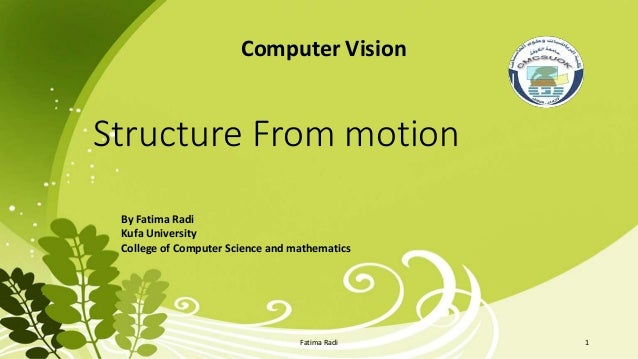Successfully reported this slideshow.Upcoming SlideShare
×

# Structure from motion

965 views

Published on

is a range imaging technique; it refers to the process of estimating three-dimensional structures from two-dimensional image sequences which may be coupled with local motion signals

Published in: Science
• Full Name
Comment goes here.

Are you sure you want to Yes NoAre you sure you want to  Yes  No
• Be the first to like this

### Structure from motion

1. 1. Structure From motion Computer Vision By Fatima Radi Kufa University College of Computer Science and mathematics 1Fatima Radi
2. 2. Outline. Introduction Review to pose estimation model How to find Essential Matrix How it can predict the locations of epipolar lines Show how to recover rotation and translation Show how to recover point positions 2Fatima Radi
3. 3. Introduction There are several ways to inferring 3D information from 2D to method so far one is • Model based pose Estimation • Stereo vision 3Fatima Radi
4. 4. Structure From motion Structure from motion (SFM) (might be better termed “structure-and- motion from a moving camera”) is a range imaging technique; it refers to the process of estimating three-dimensional structures from two-dimensional image sequences which may be coupled with local motion signals.(i.e Estimate the 3D structure and 3D (camera) motion.) 4Fatima Radi
5. 5. Review to pose estimation model Create a Scene • Create some points on the face of cube • Render image from two views 5Fatima Radi
6. 6. The Essential Matrix • The Essential Matrix is The Matrix E , That relates the Image of a point in one Camera to its image in other camera , given a translation and rotation 6Fatima Radi
7. 7. Cont. The essential matrix is E = [t]x R where • [t]x is the skew symmetric matrix corresponding to t • t is the translation of camera 2 with respect to camera 1 • R is rotation of camera 2 with respect to camera 1 7Fatima Radi
8. 8. Calculating E. E=[tx]R where [tx] is cross prodect :- [tx]= 0 − t 3 t(2) t 3 0 − t 1 −t 2 t 1 0 and general Rotation is:- R=Rx * Ry * Rz Rx=[1 0 0 ; 0 cos(x) -sin(x) ; 0 sin(x) cos(x)] Rx=[1 0 0 ; 0 cos(x) -sin(x) ; 0 sin(x) cos(x)] Rx=[1 0 0 ; 0 cos(x) -sin(x) ; 0 sin(x) cos(x)] 8Fatima Radi
9. 9. Epipolar lines • Representation of a line: • Equation of a line in the (x,y) plane is ax + by + c = 0 • line may be represented by the homogeneous coordinates l = (a,b,c)T • The point p lies on the line l if and only if pT l = 0 • l = (a,b,c)T = E p1 are the parameters of the line 9Fatima Radi
10. 10. Visualization of Epipolar lines . • Draw epipolar lines to verify that corresponding points lie on these lines • Epipolar lines • E𝑃1 is the epipolar line in the first view corresponding to P1 in the second view • 𝐸 𝑇 𝑃0 is the epipolar line in the second view corresponding to P1 in the first view • Draw the line on the first image • –Find two points (xa,ya) and (xb,yb) on the line, and draw a line between them • –Let xa = -1, solve for ya • –Let xb = +1, solve for yb • y = (– c – ax)/b 10Fatima Radi
11. 11. Recovering Motion Parameters . • once you have Essential matrix E , you can recover the relative motion between cameras • Recall that the Essential matrix is made up of the translation and rotation matrices ;ie.E = [t]x R • we can extract the translation and rotation by taking SVD of E again E = U D VT • than from the following combinations : • t is either u3 or –u3 , where u3 is the third (last) column of U • R is either U W VT or U WT VT • where W=[0 -1 0;1 0 0;0 0 1]; 11Fatima Radi